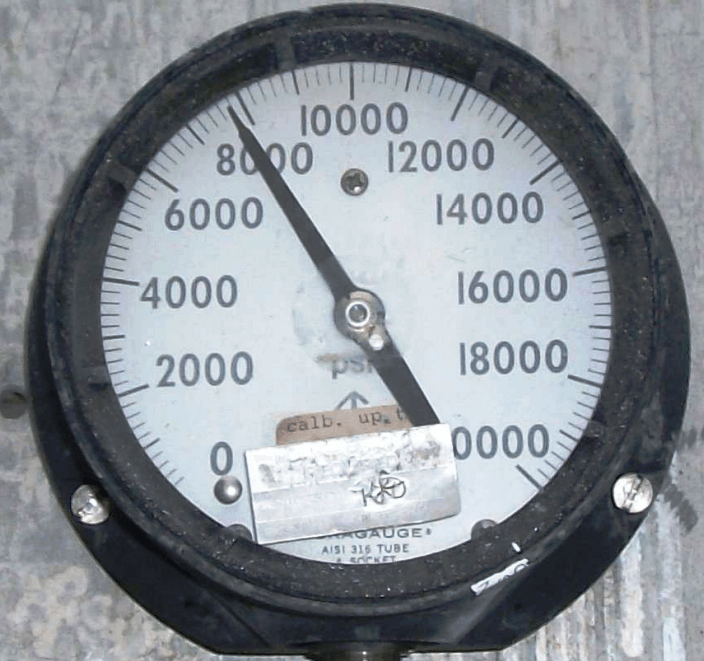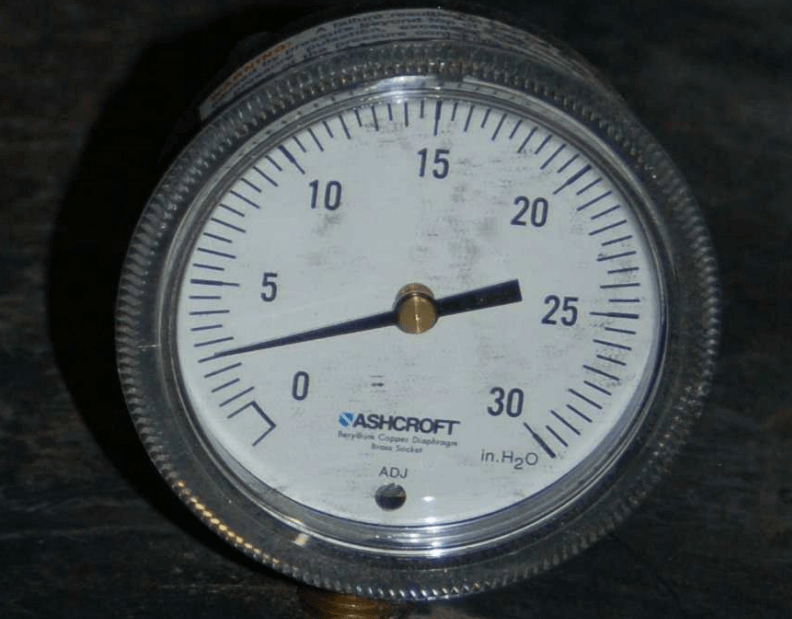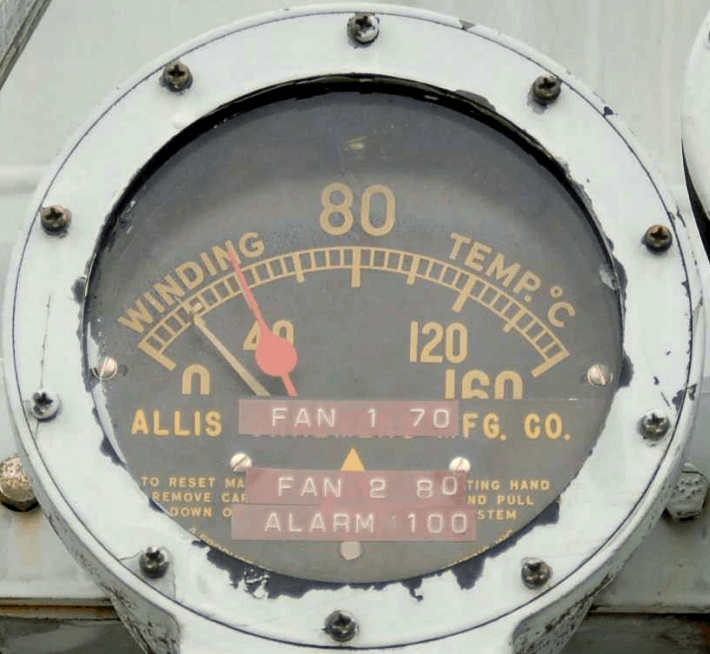# How to Read Gauge Analog Scales

#### 1. Interpret the pressure measurement displayed by this gauge mechanism, assuming a gauge accuracy of ± 1% full scale:#### How low and how high could this pressure actually be, given the stated accuracy of this gauge?

Pressure = 3750 PSI ± 60 PSI

This means the actual pressure could be as low as 3690 PSI or as high as 3810 PSI

#### 2. Interpret the pressure measurement displayed by this gauge mechanism, assuming a gauge accuracy of ± 2% full scale:#### How low and how high could this pressure actually be, given the stated accuracy of this gauge?

Pressure = 7800 PSI ± 400 PSI

This means the actual pressure could be as low as 7400 PSI or as high as 8200 PSI

#### 3. Interpret the pressure measurement displayed by this gauge mechanism, assuming a gauge accuracy of ± 0.5% full scale:#### How low and how high could this pressure actually be, given the stated accuracy of this gauge?

Pressure = 560 PSI ± 5 PSI

This means the actual pressure could be as low as 555 PSI or as high as 565 PSI.

#### 4. Interpret the pressure measurement displayed by this gauge mechanism, assuming a gauge accuracy of ± 2% full scale:#### How low and how high could this pressure actually be, given the stated accuracy of this gauge?

Pressure = 2.5 ”H2O ± 0.6 ”H2O. This means the actual pressure could be as low as 1.9 ”H2O or as high as 3.1 ”H2O.

If you look closely at the photograph, you can see that the camera’s angle to the gauge face is not straight-on, and therefore there will be some parallax error in reading this gauge’s face.

If we were to lower the camera’s view to get a more direct look at the gauge, we might see the needle pointing between the 2.5 and 3 divisions, which would mean a pressure of 2.75 ”H2O ±0.6 ”H2O.

#### 5. Interpret the pressure measurement displayed by this gauge mechanism, assuming a gauge accuracy of ± 2% full scale:#### How low and how high could this pressure actually be, given the stated accuracy of this gauge?

Pressure = 14 PSI ± 2 PSI

This means the actual pressure could be as low as 12 PSI or as high as 16 PSI.

#### 6. Interpret the temperature measurement displayed by this gauge mechanism, and also identify the meaning of the other pointer:The current temperature is 40 degrees Celsius (red pointer), and the other pointer is a low-temperature capture. In this case, the “capture” pointer shows that the temperature went down as low as 20 degrees Celsius (or perhaps a bit lower, since parallax error is making that pointer appear to read higher than it actually is).

#### 7. Interpret the measurement displayed by this Level Indicator :The red pointer shows 61% process level. The setpoint is a few percent less than that.

#### 8. Interpret the air flow measurement displayed by this rotameter:Rounding to the nearest half-division, we see the center of the ball-plummet nearest the “1” mark. No units of measurement are specified on the scale (from this viewing angle, anyway), and so we cannot say if this is 1 SCFH, 1 SCFM, or anything else.

#### 9. Interpret the pressure measurement displayed by this U-tube manometer, recalling that the pressure will be equal to the difference in heights between the two liquid columns:The pressure difference is approximately 22 inches of liquid column.

Credits : by Tony R. Kuphaldt – under CC BY 1.0

Be the first to get exclusive content straight to your email.
We promise not to spam you. You can unsubscribe at any time.
1.